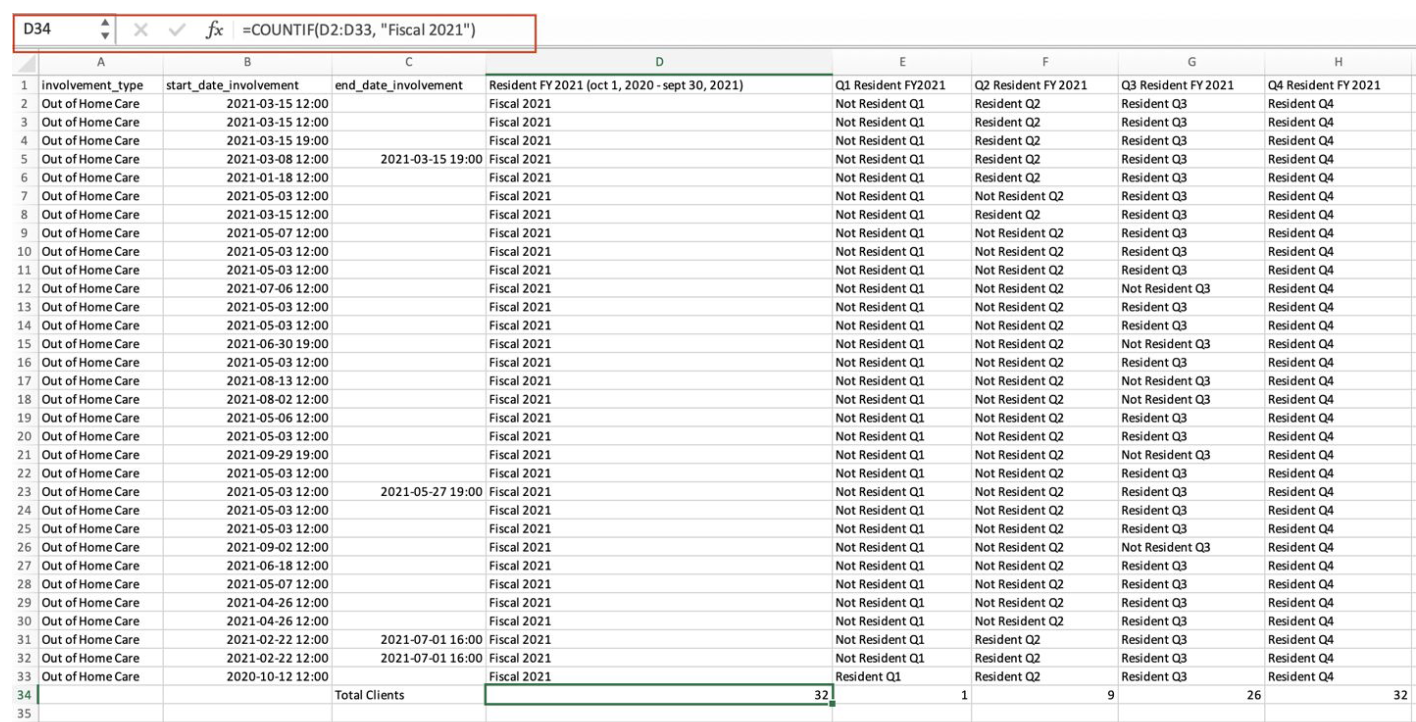# Fiscal Year Reporting: Services or Involvement Duration

## This article will cover how to use Calculated Fields to create a report that shows clients with a case involvement or enrolled in a service during a specified time period.

### How to create a report that answers the question: How many clients received a service during each quarter (or month)?

1. Create a New Visualization
2. Select the Case_Involvements Dataset
3. Change the Chart Type to Grid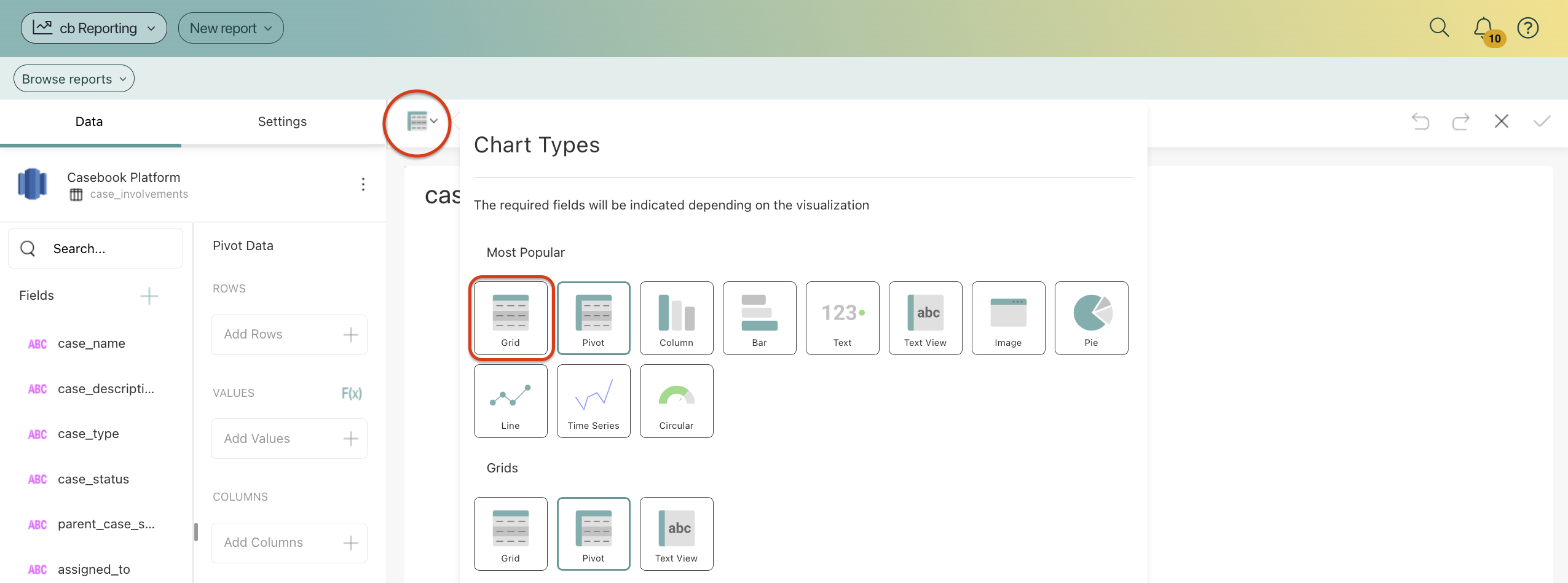4. Drag and drop the following fields into the Grid Data section under Columns
1. Involvement type
2. Involvement Start Date
3. Involvement End Date
5. Add a Filter for Involvement_Type and select the involvements that you wish to track in this report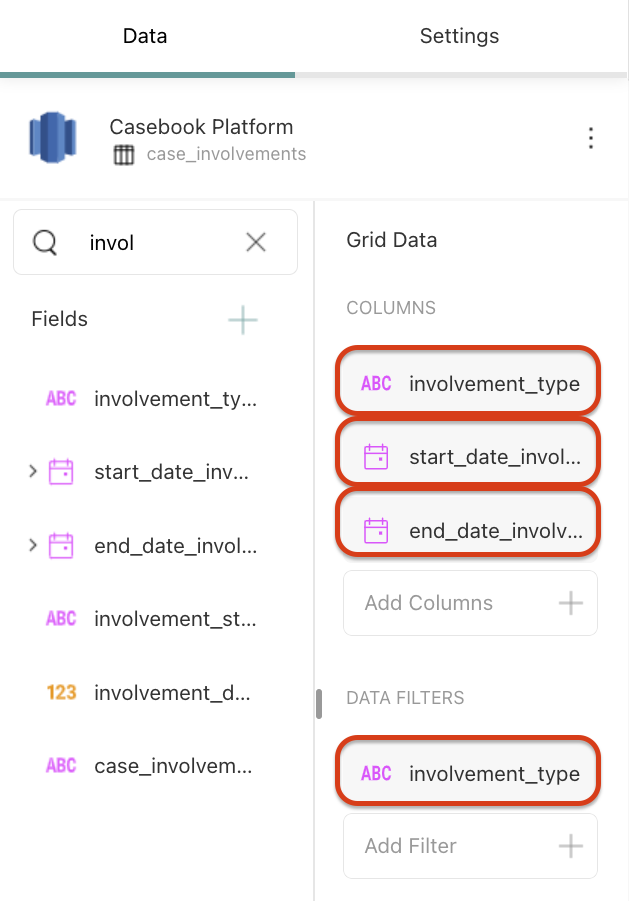6. Add a new Calculated Field by selecting the + Icon in the Fields List, then selecting Calculated Field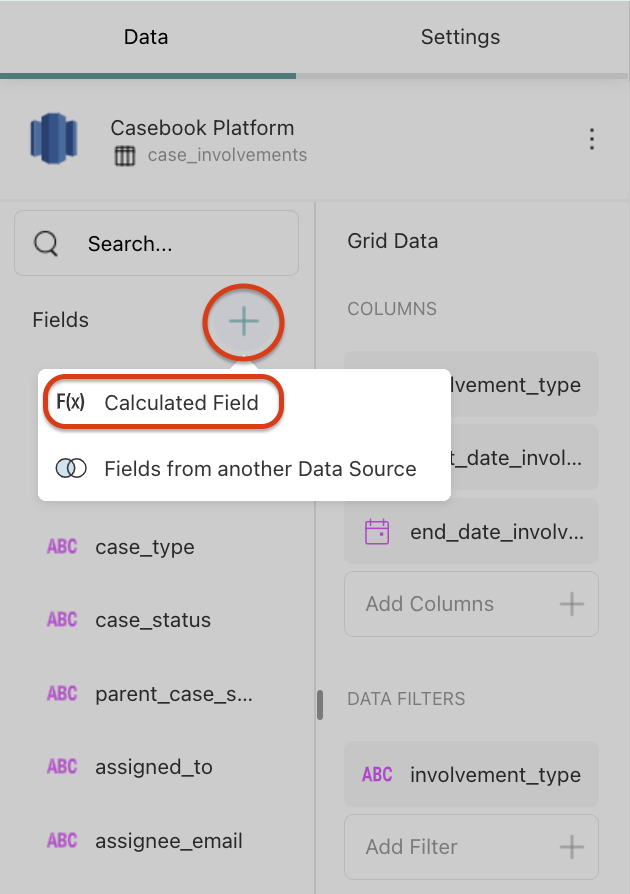7. Name the Field, then add the following formulas: Please note that you will have to repeat this process for each formula
1. FY 2022
1. if(and([start_date_involvement]> date(2021,12,31), [start_date_involvement] < date(2023,1,1)),"Fiscal 2022",if(isempty([end_date_involvement]), if(and([end_date_involvement] < date(2023,1,1), today()> date(2021,12,31)), "Fiscal 2022", "Not Fiscal 2022"),if(and([start_date_involvement] < date(2023,1,1), [end_date_involvement]> date(2021,12,31)), "Fiscal 2022", "Not Fiscal 2022")))
2.  Q1 FY22
1. if(isempty([end_date_involvement]),if(and([start_date_involvement] < date(2022,4,1),(today() > date(2021,12,31))),"Fiscal Q1","Not Fiscal Q1"),if(and([start_date_involvement] < date(2022,4,1),([end_date_involvement] > date(2021,12,31))),"Fiscal Q1","Not Fiscal Q1"))
3. Q2 FY22
1. if(isempty([end_date_involvement]),if(and([start_date_involvement] < date(2022,7,1),(today() > date(2022,3,31))),"Fiscal Q2","Not Fiscal Q2"),if(and([start_date_involvement] < date(2022,7,1),([end_date_involvement] > date(2022,3,31))),"Fiscal Q2","Not Fiscal Q2"))
4. Q3 FY22
1. if(isempty([end_date_involvement]),if(and([start_date_involvement] < date(2022,10,1),(today() > date(2022,6,30))),"Fiscal Q3","Not Fiscal Q3"),if(and([start_date_involvement] < date(2022,10,1),([end_date_involvement] > date(2022,6,30))),"Fiscal Q3","Not Fiscal Q3"))
5. Q4 FY22
1. if(isempty([service_end_date]),if(and([service_start_date] < date(2023,1,1),(today() > date(2022,9,30))),"Fiscal Q4","Not Fiscal Q4"),if(and([service_start_date] < date(2023,1,1),([service_end_date] > date(2022,9,30))),"Fiscal Q4","Not Fiscal Q4"))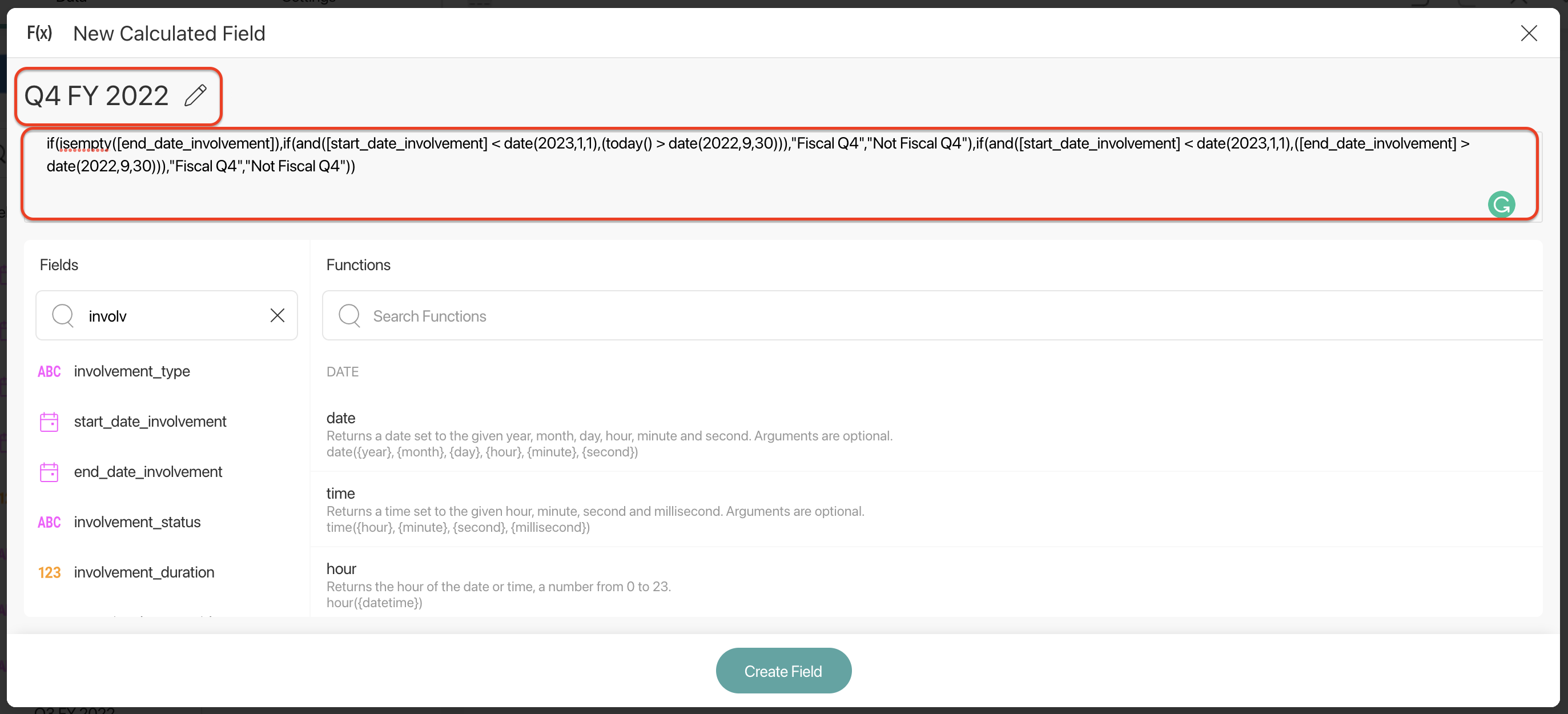8. Drag these new fields into the Grid Data section under Columns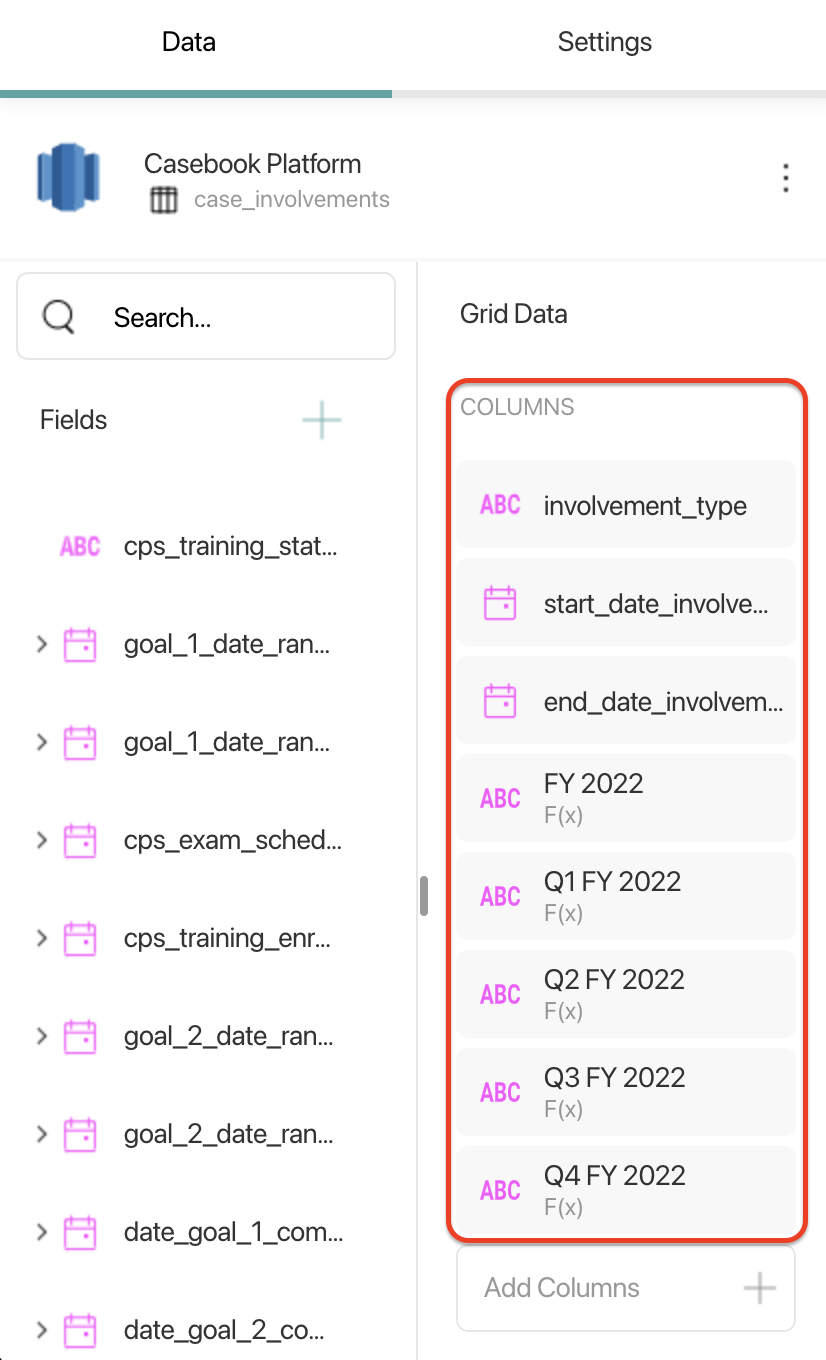9. Save the report by selecting the checkmark icon in the upper right-hand corner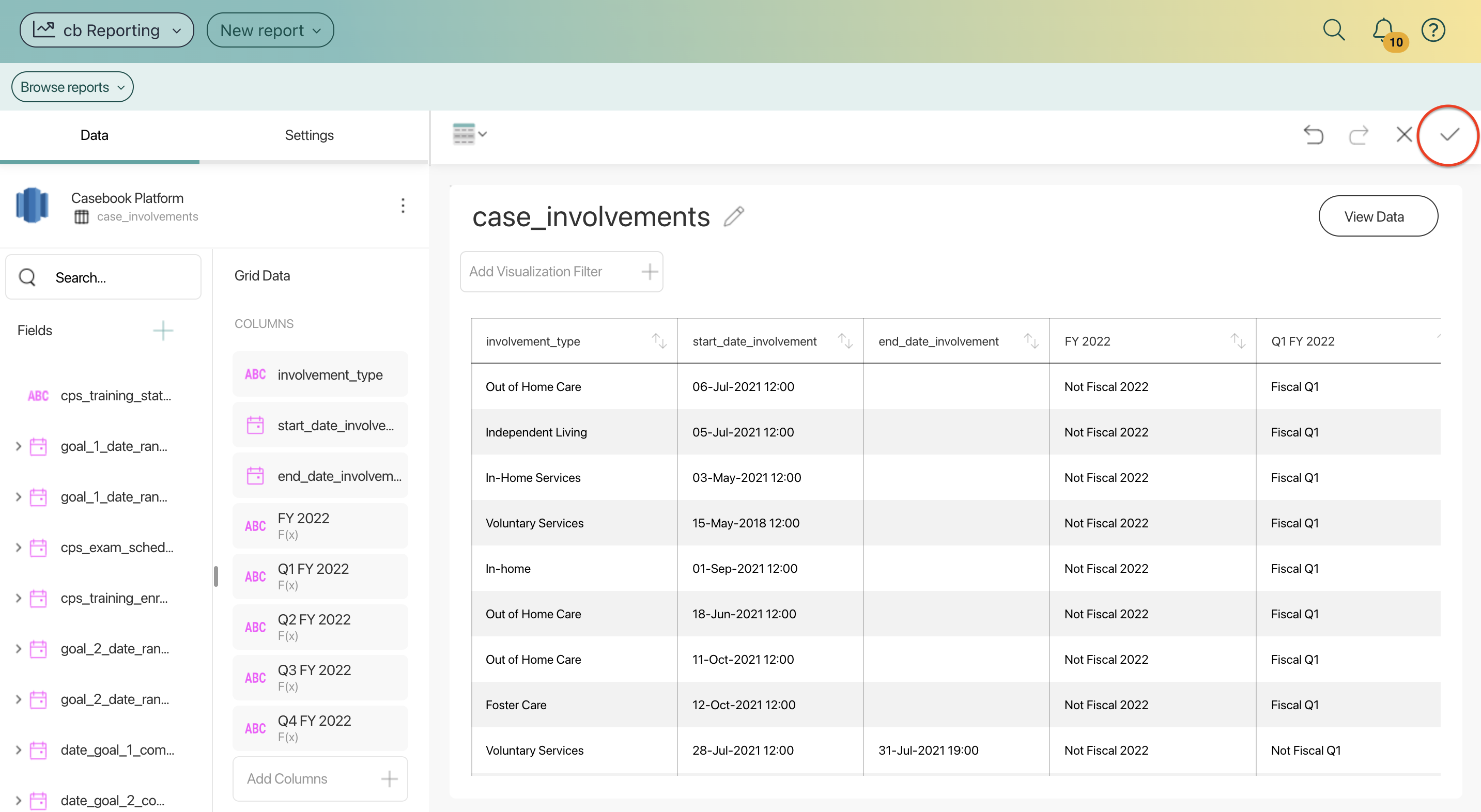10. If you would like to sum the rows, you will need to export this report to Exccel
1. At the bottom of the last row under the columns D-H use the formula below
to sum the number of residents per time range.
1. For Column D:      =COUNTIF(range, "Fiscal 2022")
2. For Column E:      =COUNTIF(range, "Fiscal Q1")
3. For Column F:      =COUNTIF(range, "Fiscal Q2")
4. For Column G:      =COUNTIF(range, "Fiscal Q3")
5. For Column H:      =COUNTIF(range, "Fiscal Q4")
11. For Column E:      =COUNTIF(range, "Fiscal Q1")